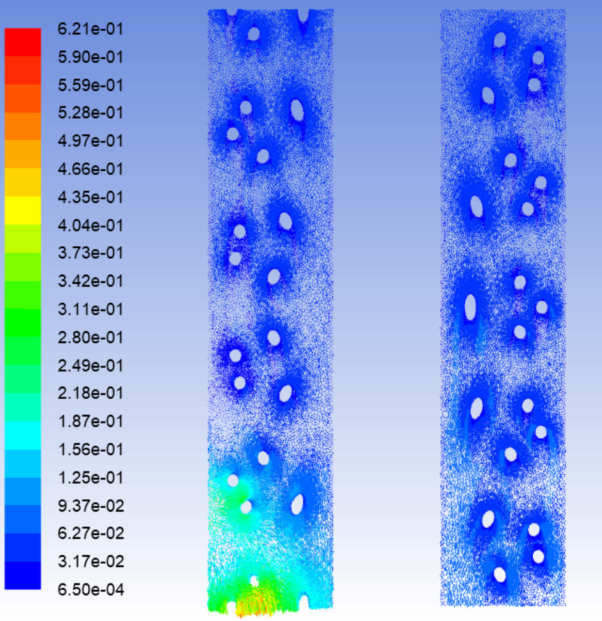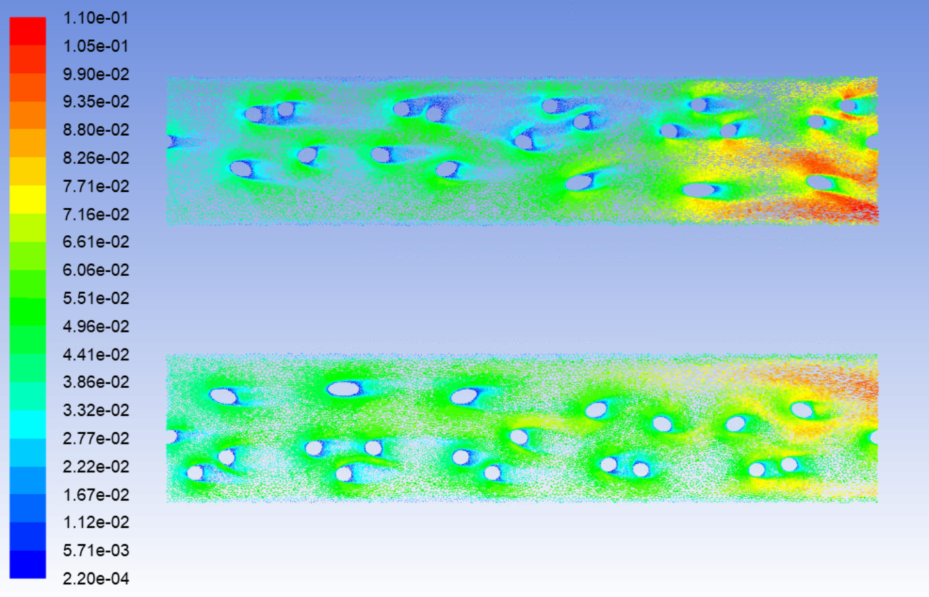ywang2524
Subscriber
Hi, I decreased the time step further to 5e-8 s and run several time steps, the overall solution does not get better. The velocity distribution on surface x=0 seems more normal, the velocity at most area is around 0.03 m/s, which is the inlet velocity. However, the velocity distribution on surface y=0 is abnormal, the velocity at most area is much smaller than the inlet velocity, and then increases sharply at the area near the outlet. Surface x=0 and surface y=0 are two vertical slices of the whole domain, why do they behave so different? Thanks so much!nvelocity distribution on surface y=0nnvelocity distribution at surface x=0nn